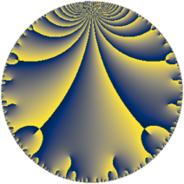# Properties

 Label 99.6.aLevel $99$ Weight $6$ Character orbit 99.a Rep. character $\chi_{99}(1,\cdot)$ Character field $\Q$ Dimension $22$ Newform subspaces $9$ Sturm bound $72$ Trace bound $2$

# Related objects

## Defining parameters

 Level: $$N$$ $$=$$ $$99 = 3^{2} \cdot 11$$ Weight: $$k$$ $$=$$ $$6$$ Character orbit: $$[\chi]$$ $$=$$ 99.a (trivial) Character field: $$\Q$$ Newform subspaces: $$9$$ Sturm bound: $$72$$ Trace bound: $$2$$ Distinguishing $$T_p$$: $$2$$

## Dimensions

The following table gives the dimensions of various subspaces of $$M_{6}(\Gamma_0(99))$$.

Total New Old
Modular forms 64 22 42
Cusp forms 56 22 34
Eisenstein series 8 0 8

The following table gives the dimensions of the cuspidal new subspaces with specified eigenvalues for the Atkin-Lehner operators and the Fricke involution.

$$3$$$$11$$FrickeDim.
$$+$$$$+$$$$+$$$$5$$
$$+$$$$-$$$$-$$$$5$$
$$-$$$$+$$$$-$$$$7$$
$$-$$$$-$$$$+$$$$5$$
Plus space$$+$$$$10$$
Minus space$$-$$$$12$$

## Trace form

 $$22q - 4q^{2} + 380q^{4} - 37q^{5} + 22q^{7} + 480q^{8} + O(q^{10})$$ $$22q - 4q^{2} + 380q^{4} - 37q^{5} + 22q^{7} + 480q^{8} - 122q^{10} - 242q^{11} - 890q^{13} - 448q^{14} + 5696q^{16} + 2840q^{17} - 1404q^{19} - 2612q^{20} + 484q^{22} + 1837q^{23} + 13745q^{25} - 2416q^{26} - 7456q^{28} + 15030q^{29} + 10579q^{31} + 3916q^{32} + 1168q^{34} + 14426q^{35} + 927q^{37} - 600q^{38} - 4668q^{40} - 43654q^{41} - 43962q^{43} - 7260q^{44} - 46786q^{46} - 58088q^{47} + 62682q^{49} + 85498q^{50} + 68432q^{52} - 3948q^{53} + 5203q^{55} + 34884q^{56} + 73908q^{58} - 23081q^{59} - 140658q^{61} - 55390q^{62} + 115552q^{64} + 114848q^{65} - 72211q^{67} + 135424q^{68} - 22940q^{70} - 105369q^{71} - 31382q^{73} - 128346q^{74} - 261528q^{76} - 38478q^{77} - 143962q^{79} - 182708q^{80} + 104260q^{82} + 195066q^{83} + 224758q^{85} - 479460q^{86} + 137940q^{88} + 49041q^{89} + 80440q^{91} + 185732q^{92} - 555160q^{94} + 249072q^{95} + 381665q^{97} + 157812q^{98} + O(q^{100})$$

## Decomposition of $$S_{6}^{\mathrm{new}}(\Gamma_0(99))$$ into newform subspaces

Label Dim. $$A$$ Field CM Traces A-L signs $q$-expansion
$$a_2$$ $$a_3$$ $$a_5$$ $$a_7$$ 3 11
99.6.a.a $$1$$ $$15.878$$ $$\Q$$ None $$-1$$ $$0$$ $$92$$ $$-26$$ $$-$$ $$+$$ $$q-q^{2}-31q^{4}+92q^{5}-26q^{7}+63q^{8}+\cdots$$
99.6.a.b $$1$$ $$15.878$$ $$\Q$$ None $$2$$ $$0$$ $$-46$$ $$148$$ $$-$$ $$+$$ $$q+2q^{2}-28q^{4}-46q^{5}+148q^{7}+\cdots$$
99.6.a.c $$1$$ $$15.878$$ $$\Q$$ None $$4$$ $$0$$ $$19$$ $$10$$ $$-$$ $$-$$ $$q+4q^{2}-2^{4}q^{4}+19q^{5}+10q^{7}-192q^{8}+\cdots$$
99.6.a.d $$2$$ $$15.878$$ $$\Q(\sqrt{33})$$ None $$-13$$ $$0$$ $$-58$$ $$146$$ $$-$$ $$-$$ $$q+(-6-\beta )q^{2}+(12+13\beta )q^{4}+(-34+\cdots)q^{5}+\cdots$$
99.6.a.e $$2$$ $$15.878$$ $$\Q(\sqrt{313})$$ None $$-1$$ $$0$$ $$38$$ $$-18$$ $$-$$ $$+$$ $$q-\beta q^{2}+(46+\beta )q^{4}+(24-10\beta )q^{5}+\cdots$$
99.6.a.f $$2$$ $$15.878$$ $$\Q(\sqrt{177})$$ None $$5$$ $$0$$ $$-58$$ $$-286$$ $$-$$ $$-$$ $$q+(3-\beta )q^{2}+(21-5\beta )q^{4}+(-34+10\beta )q^{5}+\cdots$$
99.6.a.g $$3$$ $$15.878$$ 3.3.54492.1 None $$0$$ $$0$$ $$-24$$ $$84$$ $$-$$ $$+$$ $$q-\beta _{2}q^{2}+(28-2\beta _{1}-4\beta _{2})q^{4}+(-8+\cdots)q^{5}+\cdots$$
99.6.a.h $$5$$ $$15.878$$ $$\mathbb{Q}[x]/(x^{5} - \cdots)$$ None $$-4$$ $$0$$ $$-100$$ $$-18$$ $$+$$ $$+$$ $$q+(-1+\beta _{1})q^{2}+(21-3\beta _{1}+\beta _{4})q^{4}+\cdots$$
99.6.a.i $$5$$ $$15.878$$ $$\mathbb{Q}[x]/(x^{5} - \cdots)$$ None $$4$$ $$0$$ $$100$$ $$-18$$ $$+$$ $$-$$ $$q+(1-\beta _{1})q^{2}+(21-3\beta _{1}+\beta _{4})q^{4}+\cdots$$

## Decomposition of $$S_{6}^{\mathrm{old}}(\Gamma_0(99))$$ into lower level spaces

$$S_{6}^{\mathrm{old}}(\Gamma_0(99)) \cong$$ $$S_{6}^{\mathrm{new}}(\Gamma_0(3))$$$$^{\oplus 4}$$$$\oplus$$$$S_{6}^{\mathrm{new}}(\Gamma_0(9))$$$$^{\oplus 2}$$$$\oplus$$$$S_{6}^{\mathrm{new}}(\Gamma_0(11))$$$$^{\oplus 3}$$$$\oplus$$$$S_{6}^{\mathrm{new}}(\Gamma_0(33))$$$$^{\oplus 2}$$